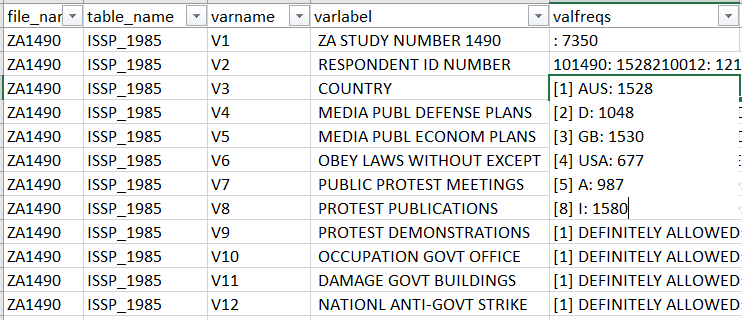# Codebook from ISSP waves 1985-2017

The International Social Survey Programme offers a wealth of data, with thematic modules repeated around every 10 years, and a solid and relatively stable block of socio-demographics. The data can be downloaded from the GESIS data archive either in separate files per year or with data bundled by topic (e.g., the Social Inequality dataset contains data from rounds 1987, 1992, 1999, and 2009).

There is no integrated codebook indicating the availability of variables in different rounds, so someone interested in longitudinal analyses would need to download all files, open them and look for the variables of interest. Like almost every long-term multi-wave survey programme, ISSP has gone through different coding and variable naming conventions, which would make the task very time-consuming.

One way to make life easier is to extract a codebook from the data, browse it, and go on to investigate in more details only the rounds that seem to have the right variables.

The codebook covers ISSP rounds from 1985 Role of Government I up to the first release of the 2017 module Social Relations and Social Networks III, the most recent file versions as of April 2019. The single wave datasets used here were downloaded by Przemek Powałko on January 2018, with the two most recent added in March 2019.

The codebook function is described in more detail in this post.

The resulting XLSX file looks like this:Creating the codebook using the code below took several hours on a laptop with a quad-core Intel i7 processor with 8 GB RAM, so it’s best to run overnight.

### loading packages
library(tidyverse) # for manipulating data
library(haven) # for opening SPSS files
library(labelled) # for using labels
library(questionr) # for getting frequencies
library(rio) # for exporting to different formats; here: XLSX

# path to folder with all ISSP data files
path <- "C:/ISSP"
temp <- list.files(path = path, pattern = "*.sav")
f <- file.path(path, temp)

issp_all <- lapply(f, haven::read_sav, user_na = TRUE)
names(issp_all) <- temp

for(i in temp) {
issp_all[[i]]$Source <- i var_label(issp_all[[i]]$Source) <- substr(i, 1, 6)
issp_all[[i]] <- issp_all[[i]] %>% select(Source, everything())
}

### codebook function
create_codebook_issp <- function(data) {
var_labels <- data.frame(cbind(names(var_label(data)), do.call(rbind, var_label(data)))) %>%
rename(varname = X1, varlabel = X2)
name_of_file <- as.character(var_labels[1,2])
freqs <- lapply(data, function(x) { return(questionr::freq(x)) }) %>%
keep(function(x) nrow(x) < 1000) %>%
do.call(rbind, .) %>%
tibble::rownames_to_column(var = "varname_value") %>%
mutate(varname = gsub("(.+?)(\\..*)", "\\1", varname_value),
value = gsub("^[^.]*.","",varname_value)) %>%
group_by(varname) %>%
mutate(npos = row_number(),
value_n = paste(value, n, sep = ": ")) %>%
select(varname, value_n, npos) %>%
mutate_at(vars(-varname), funs(ifelse(is.na(.), "", .))) %>%
unite("valfreqs", c(2:ncol(.)), sep = "\n") %>%
mutate(valfreqs = sub("\\s+\$", "", valfreqs))

full_join(var_labels, freqs, by = "varname") %>%
mutate(file_name = name_of_file) %>%
filter(varname != "Source")
}

file_names <- read.csv(paste(path, "/issp-file-names.csv", sep = ""))
issp_all_codebooks <- lapply(issp_all, create_codebook_issp)
issp_all_codebooks_df <- do.call(rbind, issp_all_codebooks) %>%
left_join(file_names) %>%
select(file_name, table_name, everything())
export(issp_all_codebooks_df, "codebook_issp_1985_2017.xlsx")